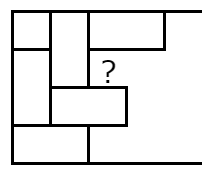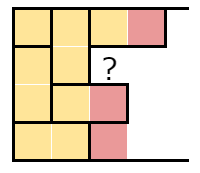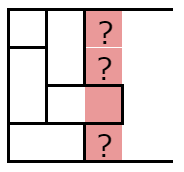Contest Duration: - (local time) (100 minutes) Back to Home

F - Hanjo 2 Editorial by en_translator

Let us denote by $$(i, j)$$ the square in the $$i$$-th column from the left and the $$j$$-th row from the top.

If $$HW$$ is small enough, one can exhaustively search all the possibility just as ABC196D “Hanjo”. How can we solve more efficiently?

$$O(2^H HW)$$ solution

Suppose that we always first determine the leftmost and the uppermost square when brute forcing.(An example of halfway-done search)

Then, all the squares above and in the left of the square we are currently focusing at are covered by tiles, and any square that is horizontally distant from the currently focused one by one or more columns is not covered.(Yellow squares: those that always covered, red squares: may or may not be covered, white squares: never covered)

Since only the red area in the illustration above is essential to the choice of covering from now on, we can define a DP (Dynamic Programming) where
$$dp[i][j][S]$$ is the number of ways of covering where every square prior to $$(i, j)$$ are already covered, and among the $$H$$ squares $$(i,j+1), \ldots,(i,H),(i+1,1),\ldots,(i+1,j)$$, the set of squares that is already covered is $$S$$.
This way, it can be solved in a total of $$O(HW2^H)$$ time.

$$O(4^H W)$$ solution

Instead of deciding one square by one, let us determine them one column by one.In such case, we can consider the following DP:
$$dp[i][S]$$ = The number of ways of covering where all the squares in the first $$(i-1)$$ columns are covered and $$S$$ is the set of covered squares among the $$H$$ squares $$(i,1),\ldots,(i,H)$$.

We will not go into details, but this DP requires transitions from $$dp[i][S]$$ to $$dp[i+1][T]$$ for all pairs $$(S,T)$$, so the time complexity is $$O(4^H W)$$.

(With some tweaks, it can even be $$O(3^H W)$$)

$$O(8^H \log W)$$ solution

The DP mentioned above has worse complexity, but one can optimize this DP to $$O(8^H \log W)$$ so that the problem is solved.

The transitions from $$dp[i][*]$$ to $$dp[i+1][*]$$ is independent of $$i$$ and is linear. Precisely, there exists a constant $$c(S,T)$$ that is only dependent on $$(S,T)$$ such that
$$dp[i+1][T]=\sum_S c(T,S) dp[i][S]$$.
Such DP can be accelerated by fast matrix exponentiation in general.

Specifically, the $$W$$-th power of the matrix where the $$(T,S)$$-element is $$c(T,S)$$, multiplied from the right by a column vector $$dp[*]$$, is a column vector $$dp[W][*]$$.

Since the size of the matrix is $$2^H \times 2^H$$, the $$W$$-th power of this can be found by fast matrix exponentiation in an $$O(8^H \log W)$$.

Since $$c(T,S)$$ can be easily constructed in an $$O(4^H H)$$ time, here the problem has been solved.

Another example problem of fast matrix exponentiation is EDPC R “Walk”.

posted:
last update: